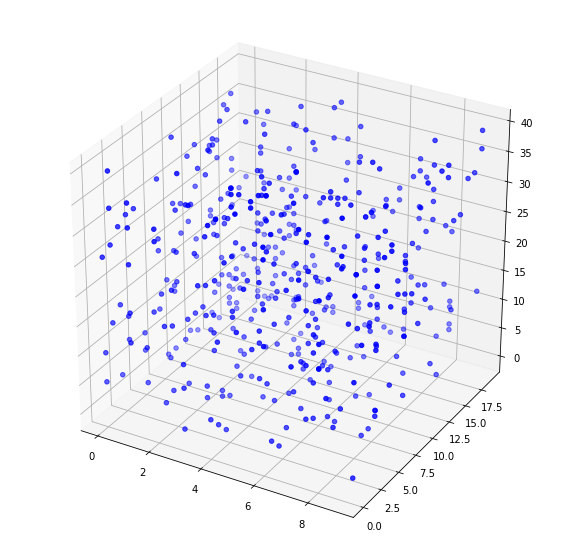Question #5855

# How to make a 3D scatter plot in Matplotlib?

Merged questions

In Python, the best way to make a 3D scatter plot is to use Matplotlib 3D scatterplot. The following example shows how to plot random points created with NumPy:

``````# Import numpy and matplotlib
import numpy as np
import matplotlib.pyplot as plt

# Creating dataset of 500 random points
x = np.random.randint(10, size =(500))
y = np.random.randint(20, size =(500))
z = np.random.randint(40, size =(500))

# Creating 3D figure
fig = plt.figure(figsize = (10, 10))
ax = plt.axes(projection ="3d")

# Plot data
ax.scatter3D(x, y, z, color = "blue")

# Show plot
plt.show()``````

.The above code should display something like:Note that scatter3D also accepts lists, the folowing will produce the same result as the above figure:

``ax.scatter3D(list(x), list(y), list(z), color = "blue")``

3 events in history

Icons proudly provided by Friconix.